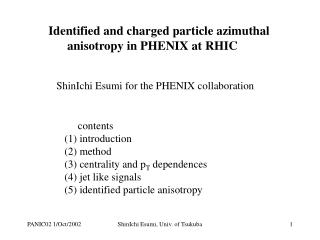DownloadDownload PresentationIdentified and charged particle azimuthal anisotropy in PHENIX at RHIC

# Identified and charged particle azimuthal anisotropy in PHENIX at RHIC

Télécharger la présentation## Identified and charged particle azimuthal anisotropy in PHENIX at RHIC

- - - - - - - - - - - - - - - - - - - - - - - - - - - E N D - - - - - - - - - - - - - - - - - - - - - - - - - - -
##### Presentation Transcript

1. Identified and charged particle azimuthal anisotropy in PHENIX at RHIC ShinIchi Esumi for the PHENIX collaboration contents (1) introduction (2) method (3) centrality and pT dependences (4) jet like signals (5) identified particle anisotropy ShinIchi Esumi, Univ. of Tsukuba

2. Target Reaction plane Projectile Reaction plane initial geometry final momentum anisotropy b:impact parameter Introduction Relation to QGP, hard processes, Jet-quenching and HBT radii, v1,v2 for different particle species and their pT, rapidity, centrality dependences ShinIchi Esumi, Univ. of Tsukuba

3. Reaction plane definition y y Reaction plane Reaction plane i 1 2 x x i plane with the directed moment plane with the elliptic moment Σ wi*sin(i) Σ wi*sin(2i) tan(1) = tan(22) = Σ wi*cos(i) Σ wi*cos(2i) (wi: 1 or pT) ShinIchi Esumi, Univ. of Tsukuba

4. reaction plane based analysis dN/d(-) = N(1 + S 2vn’cos(n(-)))  : azimuthal angle for measured particles  : reaction plane angle vn’ : raw anisotropy parameter vn =vn’/F : corrected anisotropy parameter F : reaction plane resolution pair wise correlation analysis Nreal (Df)/Nmixed(Df) = N(1 + S 2vn2cos(n(D))) Df : fi-fj F(x) = A exp(-0.5(x/s)2) + B (1+2v22cos(2x)) Gauss term is to account for some of the non-flow contribution. ShinIchi Esumi, Univ. of Tsukuba

5. dNch/dh -6 -3 0 3 6 h Beam-beam counter (BBC) |h|=3~4 64pmts in each BBC charged particles Reaction plane determination is at 3 units of rapidity away from the mid-rapidity, therefore less non-flow contributions. beam line two central arms (CNT) |h|<0.35 Dch,PCs,TOF,EMCAL tracking, momentum, PID Pair correlation analysis uses charged tracks at mid-rapidity. collision point ShinIchi Esumi, Univ. of Tsukuba

6. reaction plane correlation and resolution h = -3.5 vs h = +3.5 (directed : n=1) h = -3.5 vs h = +3.5 (elliptic : n=2) |h|=3.5 vs |h| < 0.35 (elliptic : n=2) F2(|h| = 3.5) F1(h = -3.5) F2 (h = -3.5) F1 (h = +3.5) F2 (h = +3.5) F2 (|h| < 0.35) DF = F1A- F1B DF = F2A - F2B DF = F2A - F2B <cos(DF)> <cos(2 DF)> <cos(2 DF)> A: (h = -3.5) B: (h = +3.5) A: (h = -3.5) B: (h = +3.5) A: (|h| = 3.5) B: (|h| < 0.35) charged multiplicity ShinIchi Esumi, Univ. of Tsukuba

7. v2(|h|<0.35) vs centrality v2 v2 measured at (|h|<0.35) with 3 different reaction planes at (|h|=3~4) --- statistical error --- +systematic error r.p. h = -4~-3 r.p. h = 3~4 r.p. |h| = 3~4 Au+Au at sqrt(sNN)=200GeV PHENIX Preliminary charged particles 0.2 < pT < 10 GeV/c <sin(2(f-F))> centrality (%) ShinIchi Esumi, Univ. of Tsukuba

8. v2 vs transverse momentum reaction plane based analysis (r.p. |h|=3~4) v2 pair wise correlation analysis PHENIX Preliminary error bars (1): statistics only (2): (1) + systematic (resolution) (3): (2) + systematic (back ground) Au+Au at sqrt(sNN)=200GeV min. bias pT (GeV/c) ShinIchi Esumi, Univ. of Tsukuba

9. v2 vs transverse momentum reaction plane based analysis (r.p. |h|=3~4) pair wise correlation analysis v2 PHENIX Preliminary dNg/dy=1000 dNg/dy=500 dNg/dy=200 Au+Au at sqrt(sNN)=200GeV Hydro-dynamical model (*) Hydro+pQCD (dNg/dy=1000,500,200) (**) min. bias (*) P.Huovinen, P.F.Kolb, U.W.Heinz, P.V.Ruuskanen and S.A.Voloshin, Phys. Lett. B503, 58 (2001) (**) M.Gyulassy, I.Vitev and X.N.Wang, Phys. Rev. Lett. 86, 2537, (2001) pT (GeV/c) ShinIchi Esumi, Univ. of Tsukuba

10. effect of jets on pair wise correlation (MC) Hydro-Flow HIJING Jets lead to strong anisotropy and an asymmetry Flow leads to strong anisotropy Df (deg) Df (deg) The anisotropy of the correlation function reflects both flow and Jet contribution ShinIchi Esumi, Univ. of Tsukuba

11. C() 1 2 3 0 jets like more in peripheral events and at higher pT P. Constantin PHENIX PRELIMINARY PHENIX PRELIMINARY N. N. Ajitanand Df ShinIchi Esumi, Univ. of Tsukuba

12. 20-40% Central PHENIX Preliminary PHENIX Preliminary 0.3-0.6 GeV 0.6-1 GeV 1/Ntrig dN/d 1/Ntrig dN/d PHENIX Preliminary PHENIX Preliminary 1- 2 GeV 2- 4 GeV 1/Ntrig dN/d 1/Ntrig dN/d direct measurement of jets (charged particles) with respect to triggered (survived) photon (pT > 2.5GeV/c) M. Chiu DATA PYTHIA ShinIchi Esumi, Univ. of Tsukuba

13. mass2 vs charge sign * momentum Mass2 (GeV2) charge sign * momentum (GeV/c) ShinIchi Esumi, Univ. of Tsukuba

14. Au+Au at sqrt(sNN)=200GeV v2 of identified hadrons r.p. |h|=3~4 min. bias v2 v2 (*) P.Huovinen, P.F.Kolb, U.W.Heinz, P.V.Ruuskanen and S.A.Voloshin, Phys. Lett. B503, 58 (2001) hydro model including the1st order phase transition with Tf=120MeV (*) pion kaon proton Negatives h-,pi-,K-,pbar Positives h+,pi+,K+,p PHENIX Preliminary PHENIX Preliminary pT (GeV/c) pT (GeV/c) ShinIchi Esumi, Univ. of Tsukuba

15. Au+Au at sqrt(sNN)=200GeV v2 of identified hadrons r.p. |h|=3~4 min. bias v2 v2 hydro model including the1st order phase transition with Tf=120MeV (*) pion proton (*) P.Huovinen, P.F.Kolb, U.W.Heinz, P.V.Ruuskanen and S.A.Voloshin, Phys. Lett. B503, 58 (2001) Negatives pi-&K-,pbar Positives pi+&K+,p PHENIX Preliminary PHENIX Preliminary pT (GeV/c) pT (GeV/c) ShinIchi Esumi, Univ. of Tsukuba

16. summary (1) The reaction plane based event anisotropy analysis is done at PHENIX. (2) Different techniques to extract anisotropy are compared and the results are consistent. (3) Jet signal is seen more in peripheral events and at higher pT. (4) Identified particles (p+/-,K+/-,p,pbar) v2 parameters are measured and there is clear mass dependence on v2 which is expected by a hydro-dynamical expansion. (5) There is some indication of v2(p,pbar) > v2(p+/-) for pT>2.0GeV/c, where the hydro-type model always predicts v2(p,pbar) < v2 (p+/-) ShinIchi Esumi, Univ. of Tsukuba